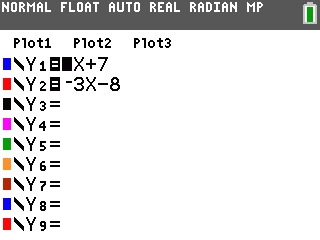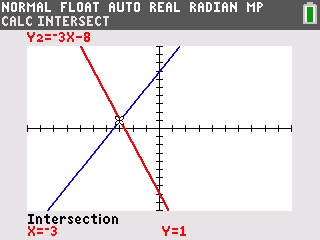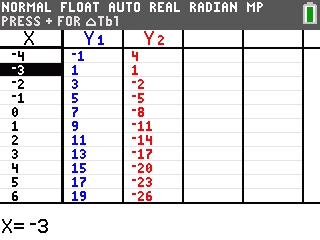# Knowledge Base

## Solution 34432: Solving a System of Linear Equations Using a Graph on the TI-84 Plus C Silver Edition Graphing Calculator.

### How do I use a graph to solve a system of linear equations on the TI-84 Plus C Silver Edition calculator?

To solve a system of linear equations using a graph on the TI-84 Plus C Silver Edition, follow the example below. The purpose is to solve a system of two equations and two unknowns.

Example: Using a graph, find the solution for the equations y = 2x + 7 and y = -3x - 8.

1) Press [Y=] to access the Y= editor.
2) For Y1, input 2X+7.
3) For Y2 input -3X-8.4) Press [GRAPH] to graph the equations.
5) Press [2nd] [TRACE]  to select the "intersect" command from the CALC menu.
6) The handheld will prompt for the "First curve" which means it wants the user to select the first equation. Press [ENTER] to mark the first equation.

Please Note: Either the Y1 equation or the Y2 equation can be marked as the first equation.

7) The handheld will prompt for the "Second curve" which means it wants the user to select the second equation. The handheld should also automatically jump to the second equation. Press [ENTER] to mark the second equation.
8) The handheld will prompt for the "Guess?" which means it wants the user to take a guess at the solution. Using the [◄] and [►] keys, move the cursor as close as possible to the intersection point (which is the solution) and press [ENTER]. The handheld will display the solutions for both the X and Y variables as a coordinate point (-3,1).9) To check the accuracy of the solution, access the TABLE by pressing [2nd] [GRAPH]. From the X column, use the [▼] and [▲] keys to scroll and locate the value (-3). When X=-3, both Y1 and Y2 are equal to 1 which means the solution is accurate.Please see the TI-84 Plus C Silver Edition guidebook for additional information.